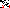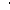# MINLPLib

### A Library of Mixed-Integer and Continuous Nonlinear Programming Instances

#### Instance st_e07

 Formatsⓘ ams gms lp mod nl osil pip py Primal Bounds (infeas ≤ 1e-08)ⓘ -400.00000000 p1 ( gdx sol ) (infeas: 0) Other points (infeas > 1e-08)ⓘ Dual Boundsⓘ -400.00000040 (ANTIGONE)-400.00000040 (BARON)-400.00000000 (COUENNE)-400.00000000 (GUROBI)-400.00000000 (LINDO)-400.00000000 (SCIP) Referencesⓘ Haverly, C A, Studies of the Behavior of Recursion for the Pooling Problem, ACM SIGMAP Bull, 25, 1978, 19-28.Tawarmalani, M and Sahinidis, N V, Convexification and Global Optimization in Continuous and Mixed-Integer Nonlinear Programming: Theory, Algorithms, Software, and Applications, Kluwer, 2002. Sourceⓘ BARON book instance misc/e07 Added to libraryⓘ 03 Sep 2002 Problem typeⓘ QCP #Variablesⓘ 10 #Binary Variablesⓘ 0 #Integer Variablesⓘ 0 #Nonlinear Variablesⓘ 3 #Nonlinear Binary Variablesⓘ 0 #Nonlinear Integer Variablesⓘ 0 Objective Senseⓘ min Objective typeⓘ linear Objective curvatureⓘ linear #Nonzeros in Objectiveⓘ 5 #Nonlinear Nonzeros in Objectiveⓘ 0 #Constraintsⓘ 7 #Linear Constraintsⓘ 4 #Quadratic Constraintsⓘ 3 #Polynomial Constraintsⓘ 0 #Signomial Constraintsⓘ 0 #General Nonlinear Constraintsⓘ 0 Operands in Gen. Nonlin. Functionsⓘ Constraints curvatureⓘ indefinite #Nonzeros in Jacobianⓘ 26 #Nonlinear Nonzeros in Jacobianⓘ 7 #Nonzeros in (Upper-Left) Hessian of Lagrangianⓘ 4 #Nonzeros in Diagonal of Hessian of Lagrangianⓘ 0 #Blocks in Hessian of Lagrangianⓘ 1 Minimal blocksize in Hessian of Lagrangianⓘ 3 Maximal blocksize in Hessian of Lagrangianⓘ 3 Average blocksize in Hessian of Lagrangianⓘ 3.0 #Semicontinuitiesⓘ 0 #Nonlinear Semicontinuitiesⓘ 0 #SOS type 1ⓘ 0 #SOS type 2ⓘ 0 Minimal coefficientⓘ 1.0000e+00 Maximal coefficientⓘ 1.6000e+01 Infeasibility of initial pointⓘ 0 Sparsity JacobianⓘSparsity Hessian of Lagrangianⓘ```\$offlisting
*
*  Equation counts
*      Total        E        G        L        N        X        C        B
*          8        6        0        2        0        0        0        0
*
*  Variable counts
*                   x        b        i      s1s      s2s       sc       si
*      Total     cont   binary  integer     sos1     sos2    scont     sint
*         11       11        0        0        0        0        0        0
*  FX      0
*
*  Nonzero counts
*      Total    const       NL      DLL
*         32       25        7        0
*
*  Solve m using NLP minimizing objvar;

Variables  x1,x2,x3,x4,x5,x6,x7,x8,x9,x10,objvar;

Positive Variables  x1,x2,x3,x4,x5,x6,x7,x8,x9;

Equations  e1,e2,e3,e4,e5,e6,e7,e8;

e1..    x1 + x2 - x3 - x4 =E= 0;

e2..    x3 - x5 + x7 =E= 0;

e3..    x4 + x8 - x9 =E= 0;

e4..  - x6 + x7 + x8 =E= 0;

e5.. x10*x3 - 2.5*x5 + 2*x7 =L= 0;

e6.. x10*x4 + 2*x8 - 1.5*x9 =L= 0;

e7.. -x10*(x3 + x4) + 3*x1 + x2 =E= 0;

e8..  - 6*x1 - 16*x2 + 9*x5 - 10*x6 + 15*x9 + objvar =E= 0;

* set non-default bounds
x1.up = 300;
x2.up = 300;
x3.up = 100;
x4.up = 200;
x5.up = 100;
x6.up = 300;
x7.up = 100;
x8.up = 200;
x9.up = 200;
x10.lo = 1; x10.up = 3;

Model m / all /;

m.limrow=0; m.limcol=0;
m.tolproj=0.0;

\$if NOT '%gams.u1%' == '' \$include '%gams.u1%'

\$if not set NLP \$set NLP NLP
Solve m using %NLP% minimizing objvar;

```

Last updated: 2023-06-01 Git hash: fdbb0df7